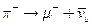Chapter 33, Problem 14PE

Chapter
Section
Textbook Problem

The primary decay mode for the negative pion isWhat is the energy release in MeV in this decay?

To determine

The energy released in the decay of π particle given by πμ+ν¯μ

Explanation

Given info:

The primary decay mode of negative pion is given by, πμ+ν¯μ

Formula used:

Energy released in a decay process = Rest energy of initial particle − sum of energies of decay products

Calculation:

In this problem the negative pion decays by πμ+ν¯μ

Rest energy of π particle =139

Still sussing out bartleby?

Check out a sample textbook solution.

See a sample solution

The Solution to Your Study Problems

Bartleby provides explanations to thousands of textbook problems written by our experts, many with advanced degrees!

Get Started

Which of the following is an example of a processed food? a. carrots b. bread c. nuts d. watermelon

Nutrition: Concepts and Controversies - Standalone book (MindTap Course List)

Write the correct IUPAC name for each of the following: a. c. b. d.

Chemistry for Today: General, Organic, and Biochemistry

The maturation of sperm takes 7080 days. ______ True ______ False

Nutrition Through the Life Cycle (MindTap Course List)